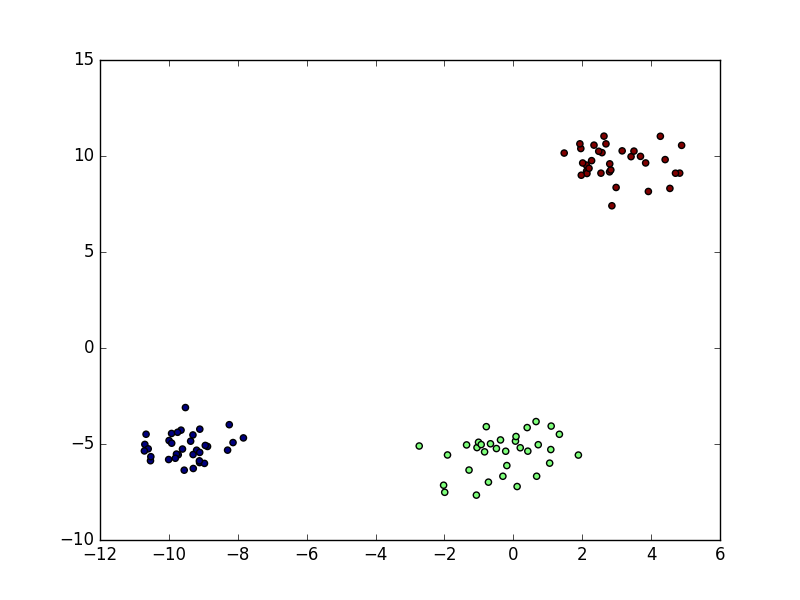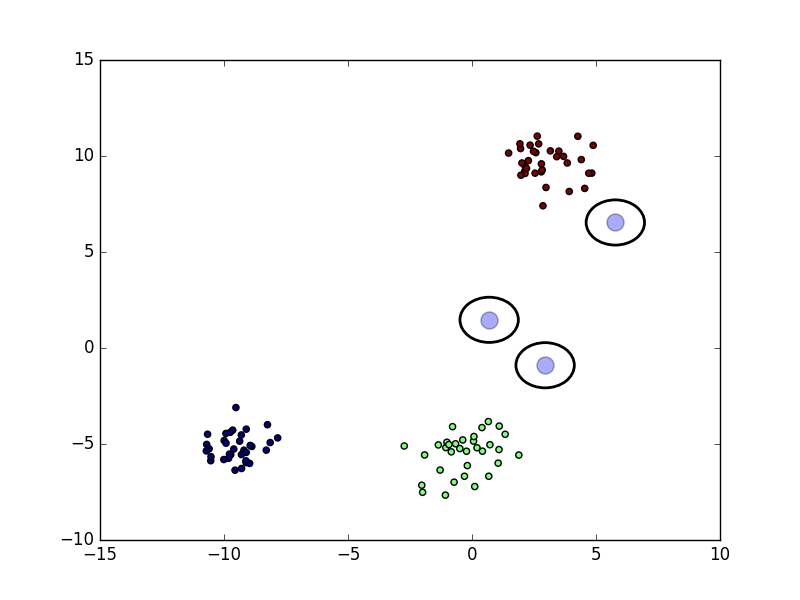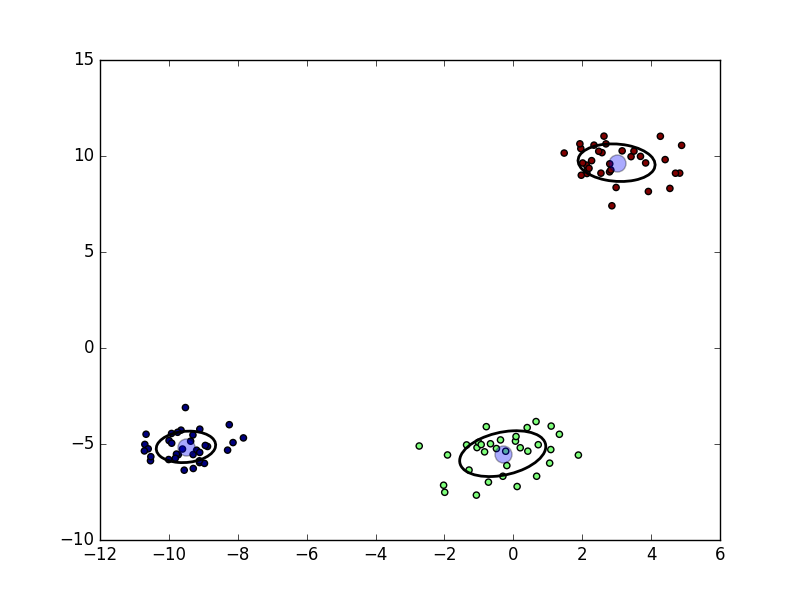2016-03-03

## Expectation Maximization in Python - Unsupervised Learning

My last post last post was about the theory of the expectation maximization algorithm. Here I provide a short and pythonic implementation for the case of a Gaussian mixture model. The first class I define is a class which represents a Gaussian component.

```class Gauss:
def __init__(self, mu, cov, pi):
self.mu = mu
self.cov = cov
self.pi = pi
self.r = None

def __repr__(self):
return "Gauss({}, {}, {})".format(self.mu, self.cov, self.pi)

def p(self, x):
return st.multivariate_normal.pdf(x, mean=self.mu, cov=self.cov)

def expectation(self, X):
self.r = self.pi * self.p(X)

def maximization(self, X):
self.maximize_pi()
self.maximize_mu(X)
self.maximize_sigma(X)

def maximize_mu(self, X):
self.mu = X.T @ self.r / self.r.sum()

def maximize_sigma(self, X):
x_minus_mu_weighted = (X - self.mu) * self.r[sp.newaxis].T
self.cov = x_minus_mu_weighted.T @ x_minus_mu_weighted / self.r.sum()

def maximize_pi(self):
self.pi = self.r.mean()
```

Next, we need a class which represents the mixture. This class implements Python's list interface. And has a fit method which calls the expectation and maximization steps.

```import scipy as sp
import scipy.stats as st

class Mixture:
def __init__(self, components):
self.components = list(components)

def __repr__(self):
return "{}({})".format(self.__class__.__name__, self.components)

def __len__(self):
return len(self.components)

def __getitem__(self, item):
return self.components[item]

def expectation(self, X):
for component in self:
component.expectation(X)
self._normalize_components()

def _normalize_components(self):
r_sum = sum(component.r for component in self)
for component in self:
component.r /= r_sum

def maximization(self, X):
for k, component in enumerate(self):
component.maximization(X)

def fit(self, X, n_steps=100):
for _ in range(n_steps):
self.expectation(X)
self.maximization(X)
```

To check if and how it works I generate a data set with scikit-learn's make_blobs. I create a mixture consisting of three components and fit it to the data.

```from sklearn.datasets import make_blobs
import matplotlib.pyplot as plt

n_classes = 3
X, y = make_blobs(n_samples=100, centers=n_classes)

mixture = Mixture(Gauss(sp.randn(2) * 4, sp.eye(2), 1 / n_classes) for _ in range(n_classes))
mixture.fit(X)
```

The original data consists of three clustersThe Gaussians are initialized randomly. At the start of the algorithm they are really offAfter 100 expectation-maximization steps they are fitted well to the data.Here, the expectation-maximization algorithm was used for unsupervised probabilistic clustering.